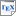[Wan06] F. Wang. Symbolic Implementation of Model-Checking Probabilistic Timed Automata. Ph.D. thesis, University of Birmingham. September 2006. [pdf] [bib] Downloads:pdf (1.03 MB)bib Notes: The source code for the implementation described in the thesis can be found here. Links: [Google] [Google Scholar] [CiteSeer] Abstract. In this thesis, we present symbolic implementation techniques for model checking probabilistic timed automata as models for systems, for example, communication networks and randomised distributed algorithms. Given a system model as probabilistic timed automata and a specification, such as, "a leader will be elected within 5 time units with probability 0.999" and "the message can be successfully delivered within 3 time units with probability 0.985", a probabilistic real-time model checker can automatically verify if the model satisfies the specification. Motivated by the success of symbolic approaches to both non-probabilistic real-time model checking and untimed probabilistic model checking, we present a symbolic implementation of model checking for probabilistic timed automata, which is based on the data structure called Multi-terminal Binary Decision Diagrams (MTBDDs). Our MTBDD-based symbolic implementation is generic with respect to the support of the real-time information. Two data structures representing the real-time information are supported, Difference Bound Matrices (DBMs) and Difference Decision Diagrams (DDDs). We develop explicit and symbolic implementations of model checking for probabilistic timed automata, focusing on probabilistic reachability properties. The explicit implementation concerns both forward and backward generation of the zone graph, where the latter results in non-convex zones in the case of calculating the minimum probability. The symbolic implementation is of the forward exploration and is built on the PRISM software. We evaluate the performance of the implementation on several realworld protocol case studies. The thesis demonstrates that model checking for probabilistic timed automata is feasible in practice and can be efficient on some real-world protocols. We conclude that to ensure efficiency of the implementation of the backward approach, support for efficient manipulation of non-convex zones and canonicity are important.